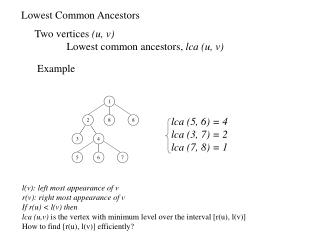DownloadDownload PresentationLowest Common Ancestors

# Lowest Common Ancestors

Download Presentation## Lowest Common Ancestors

- - - - - - - - - - - - - - - - - - - - - - - - - - - E N D - - - - - - - - - - - - - - - - - - - - - - - - - - -
##### Presentation Transcript

1. 1 2 8 8 3 4 5 6 7 Lowest Common Ancestors Two vertices (u, v) Lowest common ancestors, lca (u, v) Example lca (5, 6) = 4 lca (3, 7) = 2 lca (7, 8) = 1 l(v): left most appearance of v r(v): right most appearance of v If r(u) < l(v) then lca (u,v) is the vertex with minimum level over the interval [r(u), l(v)] How to find [r(u), l(v)] efficiently?

2. p=[5,5,3,3,3,1,1,1] s=[1,1,1,1,1,1,2,2] p=[5,5,3,3] s=[3,3,3,4] p=[7,1,1,1] s=[1,1,2,2] p=[5,5] s=[5,10] p=[3,3] s=[3,4] p=[7,1] s=[1,1] p=[8,2] s=[2,2] 5 10 3 4 7 1 8 2 Range Minima Problem Let {s1,s2,s3,s4,s5,s6,s7,s8} = {5,10,3,4,7,1,8,2} Given i, j, how to find min {ai...aj} in constant time? v1 • Approach: • Prefix min • Suffix min v3 min {ak..al} 1. Find lca w of ak and al in a complete binary tree Let x: left child, y: right child of w 2.suffix min of k in x 3. prefix min of l in y 4. take min of 2 and 3 Example {a3..a5} w = v1 suffix min of 3rd in v2 = 3 prefix min of 5th in v3 =7 min {a3..a5} = 3 v2 v4 v6 v7 v5

3. 12 4 7 9 2 6 3 4 1 5 Applications: Computing the minimum of its descendants Example For each vi, compute xi = minimum aj among all its descendants vj of vi Then xi = min {ak .. aj }, where k is preorder (vi) and j = k + descendants of (vi) -1

4. Complexity of Range Minima • Two part: • Initial construction • Searching Construction: Merging P [ i.. j] P [ j+1.. k] O ( log n ) time with O ( n ) PEs P’ [ i .. k] parent copy  Each level of three O (1) total time O (log n) time for constructing the P, S lists min (, p[j+1]) Searching: Constant time

5. 1 2 8 9 3 4 5 6 7 Breadth First Traversal level 0 level 1 Eulerian Tour ET = (v1,v2), (v2,v3), (v3,v2), (v2,v4), (v4,v5), (v5,v4), (v4,v6), (v6,v4), (v4,v7), (v7,v4), (v4,v2), (v2,v1), (v1,v8), (v8,v1), (v1,v9), (v9,v1) BFS: 1, 2, 8, 9, 3, 4, 5, 6, 7 • Remove the left most edges, and right most edges from ET • ET’ = (v1,v2), (v2,v3), (v3,v2), (v2,v4), (v4,v5), (v5,v4), (v4,v6), (v6,v4), (v4,v7), (v7,v4),(v4,v2), (v2,v1), (v1,v8), (v8,v1), (v1,v9), (v9,v1) • How to detect? • Prefix maxima, and suffix maxia of edge level • If prefix max has ai - ai-1 > 0 then i-th edge is a leftmost edge • Back Edge: “( “ Advance edge: “)” • find mate of each parenthesis • Mostly well defined • If (vi,vj) is the right most edge at level k, • then its mate is leftmost edge at level k+1 (if it exists) • (vk,vl) is a mate of (vi,vj) then make a link vk -> vi • (vi,vj) is the first edge, vi -> vj

6. Generalized Prefix Computation • Next class: read section 4.9